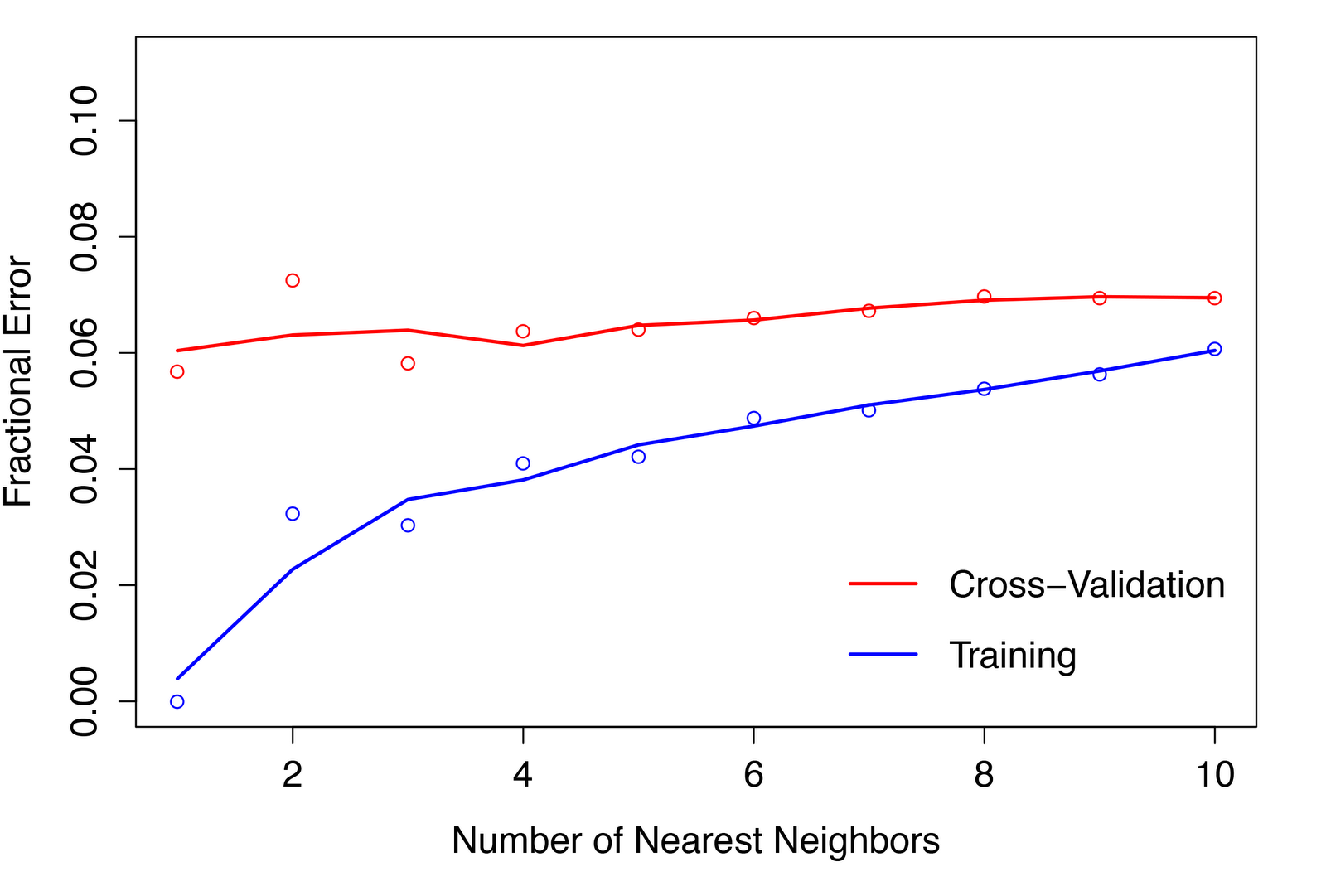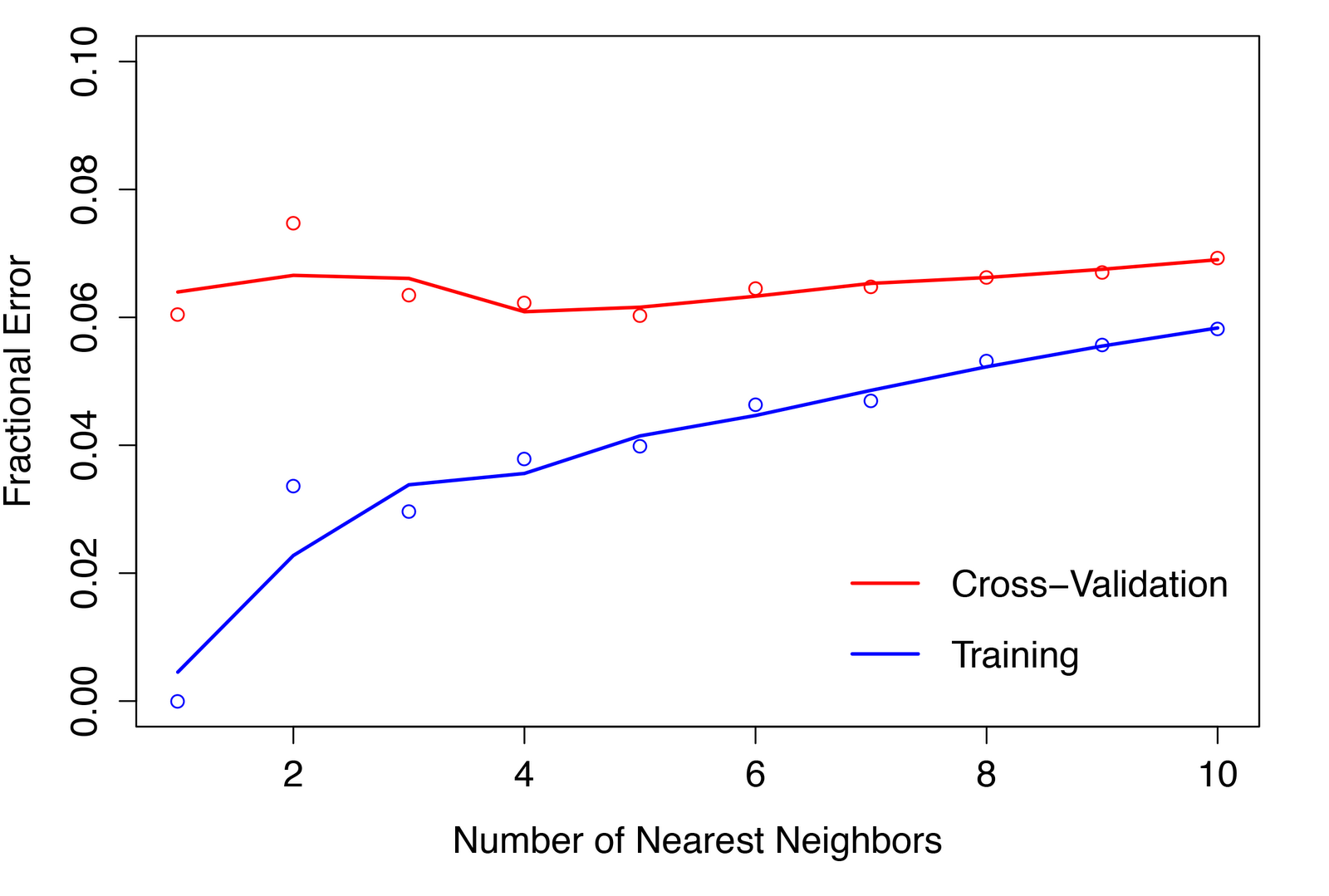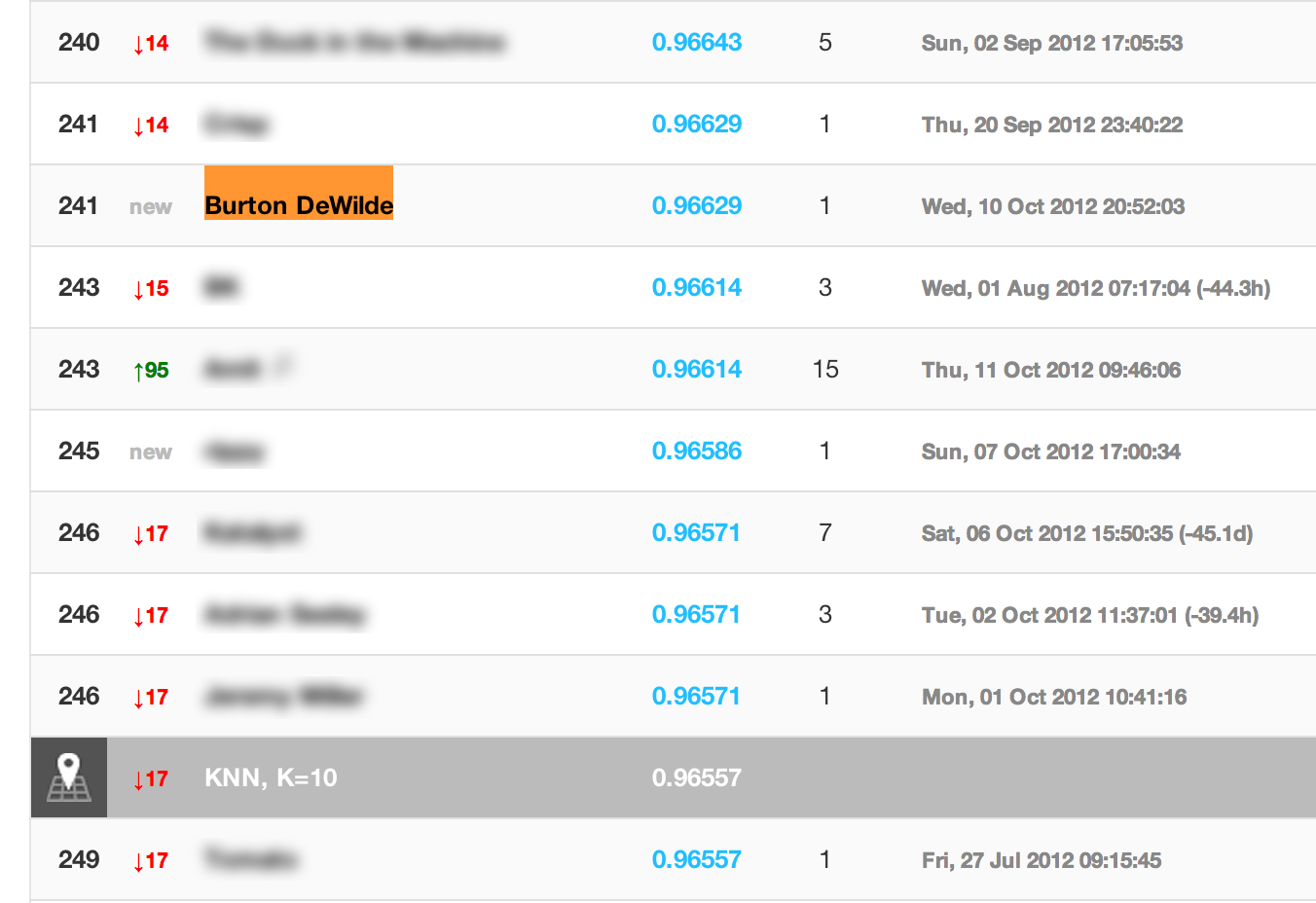Now for the fun part! In Part 1, I described the machine learning task of classification and some well-known examples, such as predicting the values of hand-written digits from scanned images. In Part 2, I outlined a general analysis strategy and visualized the training set of hand-written digits, gleaning at least one useful insight from that. Now, in Part 3, I pick a learning algorithm, train and optimize a model, and make predictions about new data!

k-Nearest Neighbor Algorithm

One of the simplest machine learning algorithms is nearest-neighbors, where an object is assigned to the class most common among the training set neighbors nearest to its location in feature-space. “Nearness” implies a distance metric, which by default is the Euclidean distance. Consider this basic example:

We have a training set consisting of two classes ($A$ and $B$) with five instances apiece, as indicated by gold and purple dots. Only two features ($x_1$ and $x_2$) are used to discriminate between the classes, so feature-space is 2-dimensional. Now, we are presented with an unlabeled observation, indicated by the red star, and would like to classify it as either $A$ or $B$. How do we do this? Well, if we assume that objects of the same class tend to be closer together in feature-space —– and, looking at the data, that assumption seems fair —– we can assign class by a majority vote of the k nearest neighbors. For the case k = 3 (small circle), one neighbor is of Class $A$ and two are of Class $B$, so we classify the unlabeled observation as a member of $B$; for k = 6 (large circle), however, four neighbors are of Class $A$ and only two are of Class $B$, so the unlabeled observation is instead classified as a member of $A$. Easy, right?

To put this mathematically:

$\hat{Y} (x) = \frac{1}{k} \sum_{x_{i} \in N_{k}(x)} y_{i}$,

where $x = (x_1, x_2)$ is the feature vector (input) for the unlabeled observation, $\hat{Y}$ is its predicted class (output), and $N_k$ is the neighborhood of $x$ defined by the k nearest neighbors $x_i$ in the training set. The prediction amounts to an average of the nearest neighbors’ classes: if $\hat{Y} > 0.5$, we classify the unlabeled observation as a member of $A$; otherwise, $B$.

Alright, let’s add a bit more data to our simple example’s training set:

We’d like to define regions in feature-space in which any unlabeled observation will be assigned to a single class ($A$ or $B$), separated by decision boundaries. Of course, the decision regions depend on the value of k, as illustrated below:

An unlabeled observation falling in the gold-colored region(s) is assigned to Class $A$; in the purple-colored region(s), Class $B$. The decision boundary, shown as a black line, corresponds to the set of positions in feature-space where $\hat{Y} = 0.5$ exactly. For k = 1 (left plot), where an unlabeled observation is given the class of its nearest neighbor, there are no misclassified training examples; however, the decision boundary is jagged and almost certainly “overtrained” to fit the training data, which means that its predictions probably won’t generalize well to new observations. For k = 25 (right plot), some training examples are misclassified, but the decision boundary is relatively smooth and seems more likely to produce reasonable predictions for new data. Clearly, choosing the right value of k for your algorithm is important; I’ll discuss how we do that later.

kNN Benchmark for Hand-written Digits

Recall that the input feature-space of the MINST hand-written digit data set is 784-dimensional, with a feature for each pixel in the 28x28 = 784-pixel images, so instead of $x = (x_1, x_2)$, we have $x = (x_1, x_2, …, x_784)$. Plus, instead of just two classes ($A$ and $B$), we have ten classes (0, 1, …, 9). Nevertheless, everything I’ve just described about kNN generalizes to this more complex classification problem. :)

A number of libraries implement kNN algorithms in R. The default is called class, but it is unacceptably slow running over large data sets because it brute-force computes the distance between an unlabeled observation and all training examples, even though most of them are far away. A faster version is in the FNN (fast nearest-neighbors) package, which uses clever data-structures like cover trees or k-d trees to reduce runtime [from O(mn) to O(m_log(_n)), where m is the number of features and n the number of training examples].

The Kaggle competition from which I got this data set provided the source code in R for a totally vanilla “benchmark” kNN model, using k = 10 and the “cover tree” algorithm for speed:

The evaluation metric used by Kaggle in this contest is classification accuracy — that is, the fraction of images in the test set that are correctly classified by the model trained on the training set. This basic implementation has a classification accuracy of 0.96557. Pretty good! And a testament to the inherent flexibility of the kNN algorithm, provided you have enough data.

# fast nearest neighbor package
library(FNN)

# training and test sets, with variable names in the first row

# split train data frame in two
# first column is class labels (0, 1, ..., 9)
labels <- train[,1]
train <- train[,-1]

# save only the knn's class predictions for each observation in test set
results <- (0:9)[knn(train, test, labels, k = 10, algorithm="cover_tree")]

# output to file as a single column of class predictions
write(results, file="knn_benchmark.csv", ncolumns=1)

The evaluation metric used by Kaggle in this contest is classification accuracy –— that is, the fraction of images in the test set that are correctly classified by the model trained on the training set. This basic implementation has a classification accuracy of 0.96557. Pretty good! And a testament to the inherent flexibility of the kNN algorithm, provided you have enough data.

Optimizing the kNN

As I wrote before, a common way to optimize the parameters of a machine learning algorithm is cross-validation, in which a fraction of the training examples is set aside (the validation set) and used like a test set — except you know the true classes for each example and can thus compare them to the model’s predictions. Rather than classification accuracy, I prefer to use classification error, i.e. the fraction of _mis_classified examples, when making these comparisons. Maybe I’m a pessimist. ;) It can be instructive to look at this error for both the training and validation sets, though you’ll want to make decisions about optimal parameters based on the cross-validation classification error.

Well. The most obvious parameter we would like to optimize is k, the number of neighbors to consider when making a prediction for an unlabeled observation. I set aside a randomly-selected 40% of the training examples for the validation set, then trained a series of models over the remaining 60% of the training set, varying the value of k from 1 to 10. I then plotted the kNN’s classification error for both the training and validation sets as a function of k:Classification error as a function of k for both training and validation sets.

You can see that the training set’s error increases with k, and for k = 1, it is exactly 0, because the single nearest neighbor to a training example is always going to be itself. As k increases, the fraction of misclassified examples increases, but it does seem to plane off a bit. Naively, I would have expected the validation set’s error to be large for small k, decrease with k up to a certain (ideal!) value, then begin to increase again. To be honest, I never resolved to my satisfaction why this wasn’t so…

… but rather than dwell on this, I decided to optimize the data as well and compare the results. Remember when we recognized that features with near-zero variance (i.e. pixels that almost always have the same value) across all classes would not be useful in discriminating between those classes? As it turns out, kNN models can get “confused” in the presence of many irrelevant features, so it’s usually a good idea to exclude those features from the model. As a bonus, it seriously reduces runtime which, as I mentioned above, goes as O(m_log(_n)). So, I simply removed those features (pixels) from the training and validation sets before feeding them into the kNN algorithm:

# helpful functions for classification/regression training
# http://cran.r-project.org/web/packages/caret/index.html
library(caret)

# get indices of data.frame columns (pixels) with low variance
print(paste("Fraction of nearZeroVar columns:", round(length(badCols)/length(train),4)))

# remove those "bad" columns from the training and cross-validation sets
train <- train[, -badCols]
cv <- cv[, -badCols]

Approximately 66% of the pixels in these images have near-zero variance, owing to the large, all-white margins around most digits. Not surprisingly, program runtime was significantly reduced, while the classification error was minimally affected:Classification error vs. k for training/validation sets, with near-zero variance features removed.

A potential pitfall of kNN models is that features with very different ranges can skew predictions, since certain features will more probably be near an unlabeled observation, while others will more probably be far. To check this, I performed feature centering and scaling — collectively, standardizing — where you subtract the mean value of a feature from each example’s value then divide by the standard deviation of the feature. So, rather than ranging from 0 to 255, pixel values are centered about 0, with +1 corresponding to a value one standard deviation above the mean, -1 as one standard deviation below the mean, and so on. Doing this in R was simple: train <- apply(train, 2, scale, center=TRUE, scale=TRUE). As it turned out, the classification error actually increased slightly:Classification error vs. k, with near-zero variance features removed and standardized features.

I’m not entirely sure why classification error should increase, but I can conceptualize why it wouldn’t decrease: All of the features (with the near-zero variance features removed) already occupy the same range (0–255), so the model predictions don’t appear to be distorted. No problem, I’ll leave feature standardization out of the model, but it was good to check.

Ultimately, I concluded that k = 5 with near-zero variance features removed gave me optimal kNN performance. I then trained a model with these parameters over the full training set (no validation set!), and ran it over the test data provided by Kaggle. How did I do compared to the benchmark shown above?Kaggle leader board at the time of my first submission.

Sweet! My model had a classification accuracy of 0.96629 —– that is, 96.629% of test observations were correctly classified —– as compared to the benchmark’s 0.96557. This placed me in about the 50% percentile of submissions, which is thoroughly mediocre but not bad for a first attempt. :)

In the next post I’ll describe one last kNN optimization and the dramatic effect it had on my model’s performance.